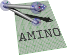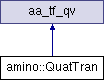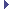amino  1.0-beta2 Lightweight Robot Utility Library
amino::QuatTran Struct Reference

A rotation quaternion and translation vector object. More...

#include <tf.hpp>

Inheritance diagram for amino::QuatTran:## Public Member Functions

QuatTran ()
Construct an idenity object.

QuatTran (const struct aa_tf_qv *S)
Construct from another quaternion-translation.

QuatTran (const struct aa_tf_qv &S)
Construct from another quaternion-translation.

QuatTran (const struct aa_tf_quat *_r, const struct aa_tf_vec3 *_v)
Construct from another quaternion-translation.

QuatTran (const struct aa_tf_quat &_r, const struct aa_tf_vec3 &_v)
Construct from another quaternion-translation.

QuatTran (const struct XAngle &_r, const struct aa_tf_vec3 &_v)
Construct from a principal angle and a vector.

QuatTran (const struct YAngle &_r, const struct aa_tf_vec3 &_v)
Construct from a principal angle and a vector.

QuatTran (const struct ZAngle &_r, const struct aa_tf_vec3 &_v)
Construct from a principal angle and a vector.

QuatTran (const struct aa_tf_duqu *S)
Construct from a dual quaternion.

QuatTran (const struct aa_tf_duqu &S)
Construct from a dual quaternion.

QuatTran (const struct aa_tf_tfmat *T)
Construct from a transformation matrix.

QuatTran (const struct aa_tf_tfmat &T)
Construct from a transformation matrix.

aa_tf_qv conj ()
Return the conjugate (inverse).

## Static Public Member Functions

static aa_tf_qv from_xyzw (double q_x, double q_y, double q_z, double q_w, double t_x, double t_y, double t_z)
Create a quaternion-translation from components.

static aa_tf_qv from_qv (const double a_r, const double a_v)
Create a quaternion-translation from a rotation quaternion and a translation vector.

static aa_tf_qv from_qv (const double e)
Create a quaternion-translation from a rotation quaternion and a translation vector.

static aa_tf_qv from_duqu (const double s)
Create a quaternion-translation from a dual quaternion.

static aa_tf_qv from_tfmat (const double t)
Create a quaternion-translation from a transformation matrix.Public Attributes inherited from aa_tf_qv
union {
struct {
aa_tf_quat_t   r

aa_tf_vec3_t   v

}

double   data 
data array

};

## Detailed Description

A rotation quaternion and translation vector object.

Definition at line 776 of file tf.hpp.

The documentation for this struct was generated from the following file: